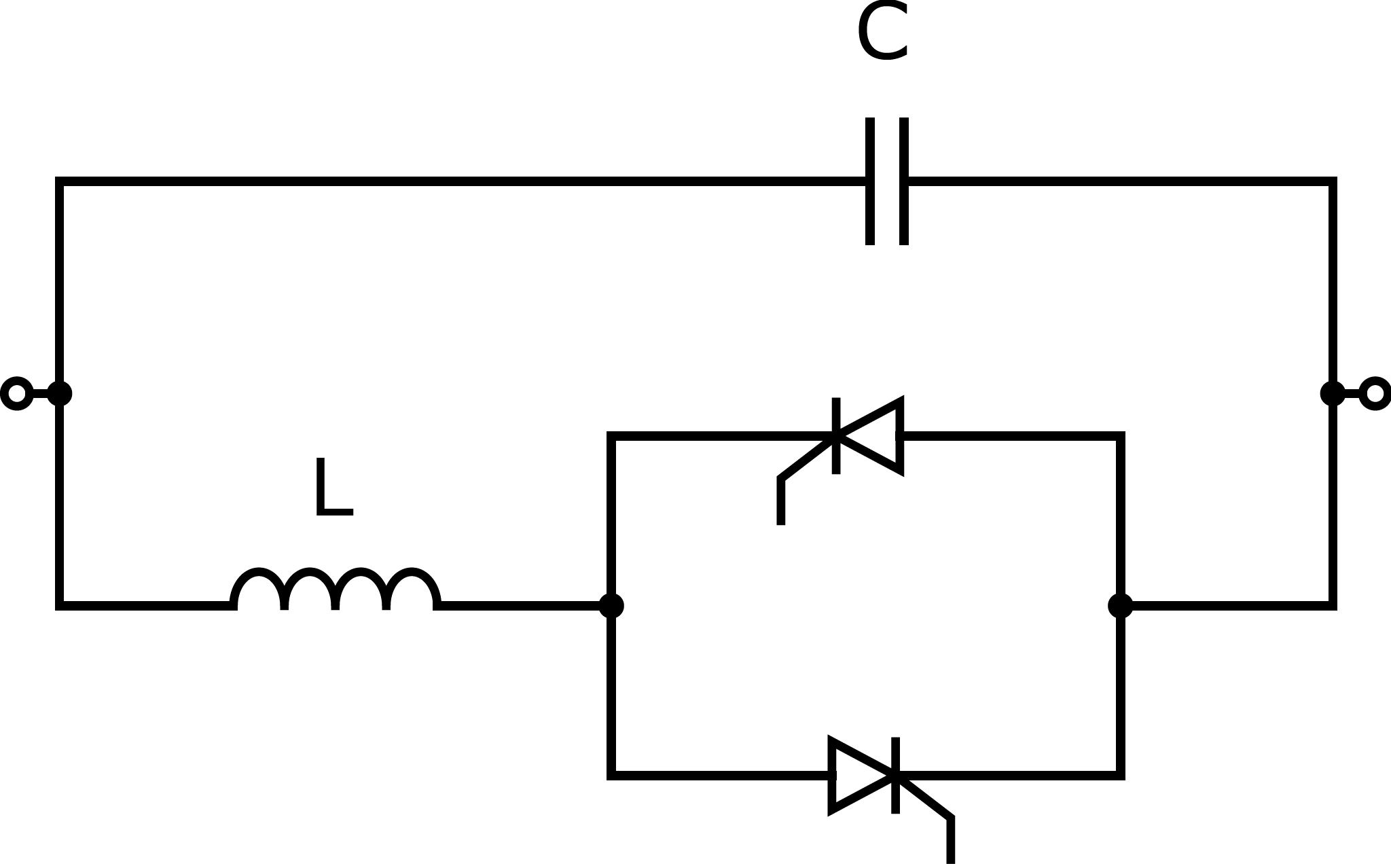# Thyristor-Controlled Series Capacitor (TCSC)

We implement the FACTS devices based on the following source:

A. Panosyan, “Modeling of advanced power transmission system controllers”, Ph.D. dissertation, Gottfried Wilhelm Leibniz Universität Hannover, 2010.

Thyristor-Controlled Series Capacitor (TCSC) device is a series element that controles the power flowing through its path by regulating its impedance. The TCSC device is very similar to the Static Var Compensator (SVC) - in fact, it is its series counterpart. Indeed, a TCSC device consists of a parallel circuit of a fixed capacitor and a reactor controlled by a pair of antiparallel thyristors. In contrast to SVC, a TCSC device is connected in series in the power system. For more context, be advised to read the documentation for the SVC elements:

We demonstrate the use-case of this device in the pandapower tutorial: FACTS.

## Create Function

pandapower.create.create_tcsc(net, from_bus, to_bus, x_l_ohm, x_cvar_ohm, set_p_to_mw, thyristor_firing_angle_degree, name=None, controllable=True, in_service=True, index=None, min_angle_degree=90, max_angle_degree=180, **kwargs)

Creates a TCSC element - series impedance compensator to control series reactance. The TCSC device allows controlling the active power flow throgh the path it is connected in.

Multiple TCSC elements in net are possible. Unfortunately, TCSC is not implemented for the case when multiple TCSC elements have the same from_bus or the same to_bus.

Note: in the Newton-Raphson power flow calculation, the initial voltage vector is adjusted slightly if the initial voltage at the from bus is the same as at the to_bus to avoid some terms in J (for TCSC) becoming zero.

min_angle_degree, max_angle_degree are placehowlders (ignored in the Newton-Raphson power flow at the moment).

INPUT:

net (pandapowerNet) - The pandapower network in which the element is created

from_bus (int) - starting bus of the tcsc

to_bus (int) - ending bus of the tcsc

x_l_ohm (float) - impedance of the reactor component of tcsc

x_cvar_ohm (float) - impedance of the fixed capacitor component of tcsc

set_p_to_mw (float) - set-point for the branch active power at the to_bus

thyristor_firing_angle_degree (float) - the value of thyristor firing angle of tcsc (is used directly if

controllable==False, otherwise is the starting point in the Newton-Raphson calculation)

OPTIONAL:

name (list of strs, None) - element name

controllable (bool, True) - whether the element is considered as actively controlling

or as a fixed series impedance

in_service (bool, True) - True for in_service or False for out of service

index (int, None) - Force a specified ID if it is available. If None, the

index one higher than the highest already existing index is selected.

min_angle_degree (float, 90) - minimum value of the thyristor_firing_angle_degree

max_angle_degree (float, 180) - maximum value of the thyristor_firing_angle_degree

OUTPUT:

index (int) - The unique ID of the created tcsc

## Input Parameters

net.tcsc

 Parameter Datatype Value Range Explanation name string name of the TCSC from_bus* integer index of the from bus where the TCSC is connected to_bus* integer index of the to bus where the TCSC is connected x_l_ohm* float $$\geq$$ 0 impedance of the reactor component of TCSC x_cvar_ohm* float $$\leq$$ 0 impedance of the fixed capacitor component of TCSC set_p_to_mw* float set-point for the power flowing through the TCSC element at the to bus thyristor_firing_angle_degree* float :math:90 leq 180 the value of thyristor firing angle of TCSC controllable* boolean True / False whether the element is considered as actively controlling or as a fixed series impedance in_service* boolean True / False specifies if the TCSC is in service. min_angle_degree float $$\geq$$ 90 minimum value of the thyristor_firing_angle_degree max_angle_degree float $$\leq$$ 180 maximum value of the thyristor_firing_angle_degree

*necessary for executing a power flow calculation.

## Electric ModelThe series impedance $$X_{TCSC}$$ of the TCSC element is calculated equivalently to SVC, according to the following equation:

\begin{align*} X_{TCSC} &= \frac{\pi X_L}{2 (\pi - \alpha) + \sin{(2\alpha)} + \frac{\pi X_L}{X_{Cvar}}} \end{align*}

The term $$X_L$$ stands for the reactance of the reactor (x_l_ohm) and the term $$X_{Cvar}$$ stands for the total capacitance (x_cvar_ohm). The thyristor firing angle $$\alpha$$ is the state variable that on the one hand defines the impedance of the element, and at the same time is the result of the Newton-Raphson calculation. The admittance $$Y_{TCSC}$$ equals $$-1j \frac{1}{X_{TCSC}}$$.

The power flow through the TCSC element is described by the following equation:

\begin{align*} \begin{bmatrix} \underline{S}_{TCSC_i} \\ \underline{S}_{TCSC_j} \end{bmatrix} = \begin{bmatrix} \underline{U}_i \\ \underline{U}_j \end{bmatrix} \begin{bmatrix} \underline{Y}_{TCSC} & -\underline{Y}_{TCSC}\\ -\underline{Y}_{TCSC} & \underline{Y}_{TCSC} \end{bmatrix}^* \begin{bmatrix} \underline{U}_i \\ \underline{U}_j \end{bmatrix}^* \end{align*}

## Result Parameters

net.res_tcsc

 Parameter Datatype Explanation thyristor_firing_angle_degree* float the resulting value of thyristor firing angle of tcsc [degree] x_ohm float resulting value of the shunt impedance of tcsc [Ohm] p_from_mw float active power consumed at the from bus of tcsc [MW] q_from_mvar float reactive power consumed at the from bus of tcsc [MVAr] p_to_mw float active power consumed at the to bus of tcsc [MW] q_to_mvar float reactive power consumed at the to bus of tcsc [MVAr] p_l_mw float active power losses of tcsc [MW] q_l_mvar float reactive power losses of tcsc [MVAr] vm_from_pu float voltage magnitude at the from bus [pu] va_from_degree float voltage angle at the from bus [degree] vm_to_pu float voltage magnitude at the to bus [pu] va_to_degree float voltage angle at the to bus [degree]